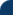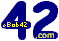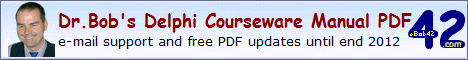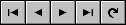Delphi Clinic C++Builder Gate Training & Consultancy Delphi Notes Weblog Dr.Bob's WebshopDr.Bob Examines... #104 See Also: Dr.Bob's Delphi Papers and ColumnsDelphi 2009 Generics
Delphi 2009 now support Generics for Win32, just like CodeGear RAD Studio 2007 supports Generics for the .NET Framework. Generics are also known as parameterised types, which actually describes more clearly what's going on: a type definition with one or more parameters (where the parameters can be types so the definition itself is considered to be a generic definition). As syntax for the parameter, Delphi uses <T> (but the T can be any identifier you wish of course).

```  type
Some<T> = array of T;
```
We can declare variables by specifying a type for the <T>, such as:
```  type
SomeIntegers = Some<integer>;

var
X: SomeIntegers;
```
This is still only a generic data definition, and adds no function. So it's really limited in its benefit.
We can also add a constraint to the generic type, stating that all instances must be at least a certain type or interface or derived thereof.
The syntax for an array of items that must be at least TComponent is as follows:
```  type
Some<T: TComponent> = array of T;
```
As a consequence, the previous defined SomeIntegers type, as Some<integer> will now no longer compile.
Instead of TComponent we can also specify TPersistent or any derived component, however we can cannot use TObject as constraint (but we can use class as constraint)

Generic Methods
Apart from Generic types, we can also define Generic method. Not generic routines, since a global procedure of function cannot be defined with a "type parameter", as the error message tells us if we try to define the following:

```  procedure Swap<AnyType>(var X,Y: AnyType);
```
However, if we add the Swap method to a class, we can implement it just fine. There are two alternatives when it comes to placing the type parameter: at the class level, or at the method level. Compare the following two definitions:
```  type
TGeneric<AnyType> = class
class procedure Swap(var X,Y: AnyType);
end;

type
TGeneric = class
class procedure Swap<AnyType>(var X,Y: AnyType);
end;
```
I prefer the latter, where I can have several class methods and can call them by passing the type parameter at the method level instead of the class level.
Note furthermore that the benefit of using class methods is that we do not need to have an actual instance of the TGeneric class (TGeneric is just a name we have to put in front).
```  class procedure TGeneric.Swap<AnyType>(var X,Y: AnyType);
var
Z: AnyType;
begin
Z := X;
X := Y;
Y := Z
end;
```
The use of this Swap method is straightforward, we just have to specify the type after the Swap, and pass the right argument variables, for example:
```  var
A,B: Integer;
begin
A := 42;
B := 17;
TGeneric.Swap<integer>(A,B);
```
We can also define other generic class methods, like an IFF (selecting either a TrueValue or a FalseValue depending on an expression) or ChooseDef (picking an item from a list, or a default value if the index is out-of-bounds):
```  type
TGeneric = class
class function IFF<AnyType>(const Expression: Boolean;
TrueValue: AnyType; FalseValue: AnyType): AnyType;
class function ChooseDef<AnyType>(index: Integer;
const values: array of AnyType): AnyType;
end;
```
The implementation of these two generic class methods is also easy:
```  class function TGeneric.IFF<AnyType>(const Expression: Boolean; TrueValue,
FalseValue: AnyType): AnyType;
begin
if Expression then Result := TrueValue
else
Result := FalseValue
end;

class function TGeneric.ChooseDef<AnyType>(index: Integer;
const values: array of AnyType): AnyType;
begin
if (index >= Low(values)) and (index <= High(values)) then
Result := values[index]
else Result := default(AnyType)
end;
```
I will show some more complex class functions, combined with anonymous methods, in another article, but let's first cover anonymous methods themselves - another new feature of Delphi 2009.

Delphi 2009 Development Essentials
This article is an excerpt from my Delphi 2009 Development Essentials courseware manual which has been sent in PDF format to all my clients, and which is sold at Lulu.com.